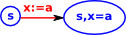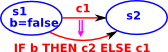# Computation Software

Here we look at some software projects and theory that relate mathematics and computation. Such as:

## ComputationComputation can be thought of a process that takes some input and uses it to produce some output.

This is like a mathematical function in that it always generates the same output for a given input (no side effects in Haskel parlance) so an example would be a sine function which generates the ratio of the opposite and hypotenuse for a given angle.

For computer theory we need to make this very general, a Turing machine can be looked at as either:

• An acceptor - accepts a recursively enumerable set.
• A generator - computes a recursive function
• An algorithm - solves yes/no problems.

So the input/output could be anything including an infinite sequence.

## Proof Assistants

More about mathematical proof on page here.

Introduction
COQ

Some sites with introduction texts:

Isabelle

## Semantics

This is concerned with 'meaning' of programs as opposed to their syntax.

## Operational SemanticsThe computations are modeled as a state with different types of command acting on that state.

There are two flavors of this big-step and small-step semantics,

#### Big-step

This tends to be a recursive process of combining the commands.

For instance, in a program we might put one command after another separated by a semicolon. So we can compose commands in a sequence defined like this:

 (c1,s1) => s2 (c2,s2) => s3 (c1;c2,s1) => s3
SequenceAn assignment (assign variable 'x' to value 'a') is more complicated because we need to do something inside the state, so this is like a second level:

 (x:=a,s1) => s(x=a)
AssignThe 'if' statement depends on the state:

 (val b,s1) (c1,s1) => s2 (IF b THEN c1 ELSE c2,s1) => s2
IfTrue(¬ val b,s1) (c1,s1) => s2 (IF b THEN c2 ELSE c1,s1) => s2
IfFalse#### Small-stepIn addition to the state we hold the commands still to be executed. This allows us to model individual steps.

iterative process
Shows how to reduce program slightly

• Abstract machine with execution state
• Reduction rules
• iterativley do reduction steps until it cant be reduced further